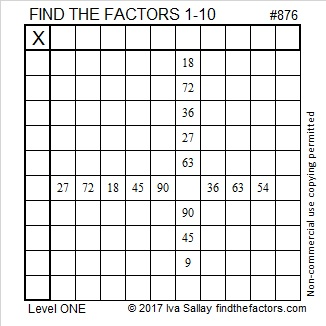# 876 and Level 1

876 consists of three consecutive numbers 6, 7, 8, so 876 has to be divisible by 3. We can also conclude the following:

• Since it’s even and divisible by 3, we know that 876 is also divisible by 6.
• Since it is divisible by 3 and it’s last two digits are divisible by 4, we know that 876 is also divisible by 12.Print the puzzles or type the solution on this excel file: 10-factors-875-885

876 is a palindrome in four other bases:

• 727 BASE 11, because 7(121) + 2(11) + 7(1) = 876
• 525 BASE 13, because 5(13²) + 2(13¹) + 5(13º) = 876
• 282 BASE 19, because 2(19²) + 8(19¹) + 2(19º) = 876
• 1A1 BASE 25 (A is 10 base 10), because 1(25²) + 10(25¹) + 1(25º) = 876

876 is also the hypotenuse of Pythagorean triple, 576-660-876 which is 12 times (48-55-73).

• 876 is a composite number.
• Prime factorization: 876 = 2 × 2 × 3 × 73, which can be written 876 = 2² × 3 × 73
• The exponents in the prime factorization are 2, 1, and 1. Adding one to each and multiplying we get (2 + 1)(1 + 1)(1 + 1) = 3 × 2 × 2 = 12. Therefore 876 has exactly 12 factors.
• Factors of 876: 1, 2, 3, 4, 6, 12, 73, 146, 219, 292, 438, 876
• Factor pairs: 876 = 1 × 876, 2 × 438, 3 × 292, 4 × 219, 6 × 146, or 12 × 73,
• Taking the factor pair with the largest square number factor, we get √876 = (√4)(√219) = 2√219 ≈ 29.597297This site uses Akismet to reduce spam. Learn how your comment data is processed.